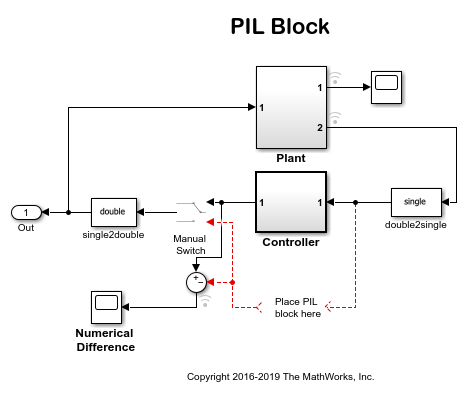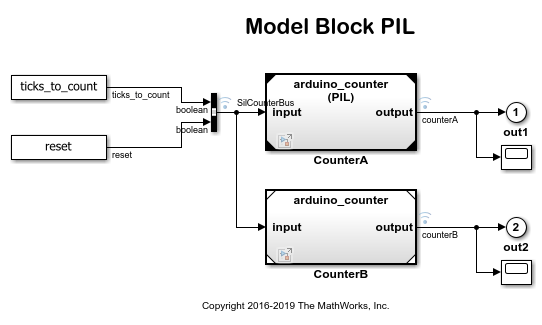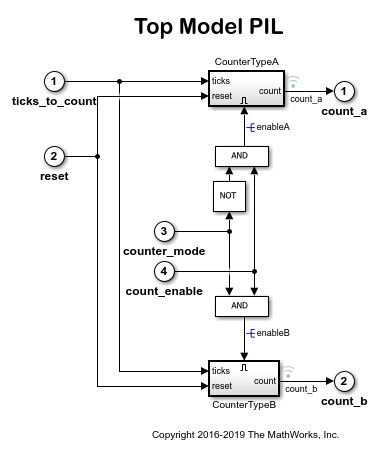Documentation

## Code Verification and Validation with PIL

This example shows you how to use Simulink Support Package for Arduino Hardware for code verification and validation using PIL.

Supported Hardware:

• Arduino Mega 2560

• Arduino Due

• Arduino Uno

• Arduino Nano 3.0

• Arduino MKR1000

• Arduino MKR WIFI 1010

• Arduino MKR ZERO

The provided models are pre-configured for Arduino Mega 2560 and can be run on any of the board listed in the "Supported Hardware" section, by changing the "Hardware board" parameter in the configuration parameters dialog box of the model as described in Task 1 of this example.

### Introduction

In this example you will learn how to configure a Simulink model to run Processor-In-the-Loop (PIL) simulation. In a PIL simulation, the generated code runs on target hardware. The results of the PIL simulation are transferred to Simulink to verify the numerical equivalence of the simulation and the code generation results. The PIL verification process is a crucial part of the design cycle to ensure that the behavior of the deployment code matches the design.

This example introduces the Simulink code generation and verification workflow by showing you how to:

• Configure a Simulink model to run PIL simulations on the Arduino hardware.

### Prerequisites

Before you start with this example, we recommend you complete the Getting Started with Arduino® Hardware example.

### Task 1 - Configure the Model for Supported Arduino Hardware

In this task, you will configure the model for the supported Arduino board.

2. In your Simulink model, click Simulation > Model Configuration Parameters to open Configuration Parameters dialog.

3. Select the Hardware Implementation pane and select your required Arduino hardware from the Hardware board parameter list. Do not change any other settings.

4. Click OK.### Task 2 - Verify the generated code for a subsystem using a PIL block

This example shows how to use a PIL block for subsystem code verification. With this approach:

• You can verify the code generated for a subsystem

• You must provide a test harness model to supply test vector or stimulus inputs

• You must swap your original subsystem with a generated PIL block; you should be careful to avoid saving your model in this state as you would lose your original subsystem

1. Open the PIL Block model. This model is configured for Arduino hardware. The objective here is to create a PIL block out of the Controller subsystem that you will run on the Arduino hardware.

2. Follow the below steps to enable PIL:3. Follow the below steps to create a PIL block for the Controller subsystem:4. Follow the below steps to run the PIL simulation:5. You can switch between the original and PIL block subsystems by double clicking on the Manual Switch block. Double click on the Numerical Differences block to see the difference between the simulated Controller subsystem and the PIL block running on the hardware.### Task 3 - Verify referenced model code using PIL

This example shows how to verify the generated code for a referenced model by running a PIL simulation. With this approach:

• You can verify code generated for referenced models

• You must provide a test harness model to provide a test vector or stimulus inputs

• You can easily switch a Model block between normal and PIL simulation mode

1. Open the Model Block PIL model. This model is configured for Arduino hardware. The model contains two Model blocks that both point to the same referenced model. You will configure one Model block to run in PIL mode while the other one will be set to normal mode.

2. Follow the below steps to configure and run the CounterA Model block in PIL mode.3. When the model starts running, Scope1 displays the PIL simulation output running on the target hardware while Scope2 shows the normal mode simulation output.### Task 4 - Verify top model code using PIL

This example shows how to verify the generated code for a model by running a PIL simulation. With this approach:

• You can verify code generated for a top model

• You must configure the model to load test vectors or stimulus inputs from the MATLAB workspace

• You can easily switch the entire model between normal and PIL simulation mode

1. Open the Top Model PIL model. This model is configured for Arduino hardware.

2. Follow the below steps to run the top model PIL simulation:3. When the PIL simulation terminates, a logsOut variable is created in the base workspace. The logsOut data contains PIL simulation results. You can access the logged data for signals count_a and count_b using the following commands:

• count_a = get(logsOut,'count_a');

• count_a.Values.Data

• count_b = get(logsOut,'count_b');

• count_b.Values.Data

### Summary

This example introduced code verification workflow using PIL simulation.